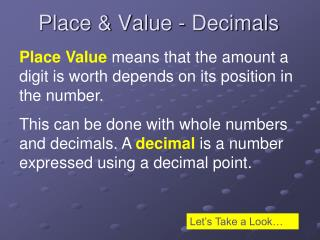DownloadDownload PresentationPlace & Value - Decimals

# Place & Value - Decimals

Download Presentation## Place & Value - Decimals

- - - - - - - - - - - - - - - - - - - - - - - - - - - E N D - - - - - - - - - - - - - - - - - - - - - - - - - - -
##### Presentation Transcript

1. Place Value means that the amount a digit is worth depends on its position in the number. This can be done with whole numbers and decimals. A decimal is a number expressed using a decimal point. Place & Value - Decimals Let’s Take a Look…

2. Decimals Whole Numbers Tens Ones Hundreds Tenths Thousandths Hundredths The very important little decimal point

3. Don’t forget those “th”s in your Dethimals! This shows a tenTH, because it takes 10 of them to make a whole This shows a hundredTH, because it takes 100 of them to make a whole If this represents a whole, or “one” then…

4. Confused by decimals? Think of them like money… This shows a tenTH, because it takes 10 of them to make a whole This shows a hundredTH, because it takes 100 of them to make a whole If this represents a whole, or “one” then…

5. By using the place value chart, we can determine the place and value of any digit within a number. Hundred Tens Hundredth Thousandth Ones Tenth Decimals Ones What very important little thing is missing here? Yes! Our very important friend, the decimal point!

6. Write a decimal for the model shown. 2 4 Hundredth Thousandth Ones Tenth Decimals

7. Write a decimal for the model shown. 8 Hundredth Thousandth Ones Tenth Decimals

8. Write a decimal for the model shown. 1 2 Hundredth Thousandth Ones Tenth Decimals

9. Let’s try using the chart! 8 3 7 2 Hundred Tens Hundredth Thousandth Ones Tenth Ones Decimals What is the place of the digit 3?Tenths What is the value of the digit 8? 8 What is the place of the digit 2? Thousandths What is the place of the digit 7? Hundredths What is the value of the digit 7? .07

10. Expanded Form with Decimals Expanded Form means writing a number as the sum of the value of its digits. You handle expanded form with decimals the exact same way you do with whole number—break apart each digit’s value and connect them with addition! Let’s Try a Few…

11. 13.875 in expanded form is: 10 + 3 + .8 + .07 + .005 23.405 in expanded form is: 20 + 3 + .4 + .005 ***Notice in this example, there is a zero in the “hundredths” place, so we can just leave it out of our expanded notation!*** 607.094 in expanded form is: 600 + 7 + .09 + .004 Ready to try some on your own?

12. Try “expanding” these numbers! 305.765 99.945 4.006 25.002 .093 123.405 513.076 34.661

13. When we compare and order decimals we do it just like we do with whole numbers: we always start from the digit on the left and move to the right—just like the direction you read! • Don’t forget the golden rule of place value work: Line Up, or… Comparing Decimals MESS UP!!!

14. Remember, if the numbers you are comparing don’t don’t have the same number of digits, you can always add a zero as a place holder! 2.003 12.103 0 Remember: Line them up, then start from the left and compare each digit!

15. .809 .810 41.203 400.102 6.320 6.317

16. Put these numbers in order from Least to Greatest! Remember: The most common mistake students make is reversing the order of least to greatest and vice versa! Don’t fall in that trap! Check and re-check yourself! 13.43 13.33 14.03 6.09 5.99 6.91 2.32 3.31 .484

17. Put these numbers in order from Greatest to Least! 745.505 545.955 744.355 9.080 9.800 9.900 1.230 .999 2.310

18. 5.603 Which number has 6 in the thousandths place? 6.600 6.536

19. Which of the following shows eighty-nine and seventy-two hundredths? 89.72 897.2 89.072

20. Which number is read seventy-one and eighteen hundredths? 71.018 71.18 7.118

21. 0.105 > 0.15 Which of the following is true? 0.05 < 0.015 .005 < .100

22. 40.000 What is 35.042 rounded to the nearest ten? 35.000 30.000

23. 5.890 What is 5.892 rounded to the nearest tenth? 5.900 6.000

24. 300.000 What is 253.765 rounded to the nearest hundredth? 253.770 253.800